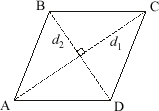# area of a rhombus

Area of a Rhombus

Formulas are given below. Note that the diagonals of a rhombus are perpendicular (as is the case with all kites).

 Rhombuss = side length of rhombush = height of rhombusd1 = long diagonal of rhombusd2 = short diagonal of rhombusArea = hs         = s2 sin A         = s2 sin B         = (½)d1d2